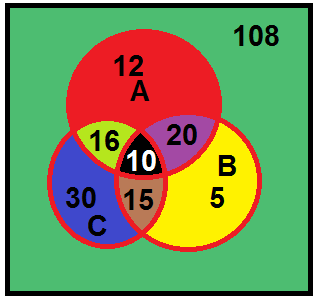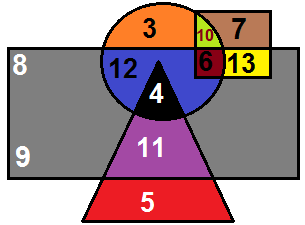# Venn Diagrams (Set Theory)Observations from the diagram
1.    Milk(A) but not Tea(B) = Only Milk(A) = 7
2.    A ∩ B’ = Only A = 7
3.    A – B = Only A = 7
4.    A ∩ B = Common in A & B = 7
5.    A B = A & B Together = 7+5+8= 20

Standard results:
1.    n(A ∪ B) = n(A) +n(B) – n(A ∩ B)
2.    n(A ∪ B ∪ C) = n(A) +n(B) +n(C) – n(A ∩ B) – n(B ∩ C) – n(A ∩ C) + n(A ∩ B ∩ C)
Example:Observations:
1.    n(A ∪ B ∪ C) = 108
2.    n(A ∪ B)         = 108-30= 78
3.    A ∩ B            = 20+10= 30
4.    A & B but not C = A ∩ B ∩ C’ = 20
5.    A ∩ C ∩ B’ = 16
6.    B ∩ C  ∩ A’ =15
7.    A ∩ B’ ∩ C’ = Only A= 12

Example:Circle- Urban
Square- Civil Servants
Rectangle- Males
Triangle- Educated

Observations:
1.    Who among the following is neither a civil servant nor educated but urban and not male---3
2.    Who among the following is a female urban residence and also a civil sevant-----10
3.    Total number of only males--- 14+9+8= 31Thanks for reading Venn Diagrams (Set Theory)

←Previous
« Prev Post
Next→
Next Post »

Video Lectures